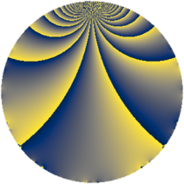# Properties

 Label 3630.2.fLevel $3630$ Weight $2$ Character orbit 3630.f Rep. character $\chi_{3630}(3629,\cdot)$ Character field $\Q$ Dimension $216$ Sturm bound $1584$

# Related objects

## Defining parameters

 Level: $$N$$ $$=$$ $$3630 = 2 \cdot 3 \cdot 5 \cdot 11^{2}$$ Weight: $$k$$ $$=$$ $$2$$ Character orbit: $$[\chi]$$ $$=$$ 3630.f (of order $$2$$ and degree $$1$$) Character conductor: $$\operatorname{cond}(\chi)$$ $$=$$ $$165$$ Character field: $$\Q$$ Sturm bound: $$1584$$

## Dimensions

The following table gives the dimensions of various subspaces of $$M_{2}(3630, [\chi])$$.

Total New Old
Modular forms 840 216 624
Cusp forms 744 216 528
Eisenstein series 96 0 96

## Trace form

 $$216q - 216q^{4} - 16q^{9} + O(q^{10})$$ $$216q - 216q^{4} - 16q^{9} + 8q^{15} + 216q^{16} - 4q^{25} - 48q^{31} + 8q^{34} + 16q^{36} + 48q^{45} + 256q^{49} - 8q^{60} - 216q^{64} - 32q^{69} + 24q^{70} + 32q^{75} - 96q^{81} - 96q^{91} + O(q^{100})$$

## Decomposition of $$S_{2}^{\mathrm{new}}(3630, [\chi])$$ into newform subspaces

The newforms in this space have not yet been added to the LMFDB.

## Decomposition of $$S_{2}^{\mathrm{old}}(3630, [\chi])$$ into lower level spaces

$$S_{2}^{\mathrm{old}}(3630, [\chi]) \cong$$ $$S_{2}^{\mathrm{new}}(165, [\chi])$$$$^{\oplus 4}$$$$\oplus$$$$S_{2}^{\mathrm{new}}(330, [\chi])$$$$^{\oplus 2}$$$$\oplus$$$$S_{2}^{\mathrm{new}}(1815, [\chi])$$$$^{\oplus 2}$$